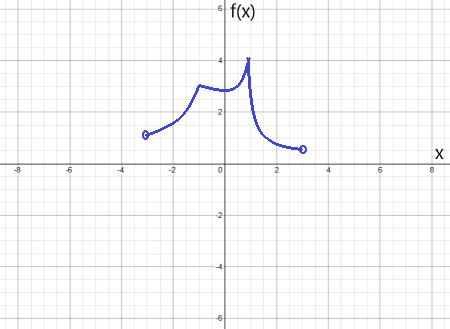# For the following exercise, draw a graph that satisfies the given specifications for the domain x...

## Question:

For the following exercise, draw a graph that satisfies the given specifications for the domain {eq}x = (-3,3) {/eq}. The function does not have to be continuous or differentiable.

There are local maxima at {eq}x = \pm 1 {/eq}, the function is concave up for all {eq}x {/eq}, and the function remained positive for all {eq}x {/eq}.

## Graphs of Functions that are Concave Up or Concave Down:

If a function is concave up at a given point then the graph of the function near that point lies above the line tangent to that point. Likewise, if a function is concave down at a given point then the graph of the function near that point lies below the line tangent to that point.

Draw a graph that satisfies the specifications: